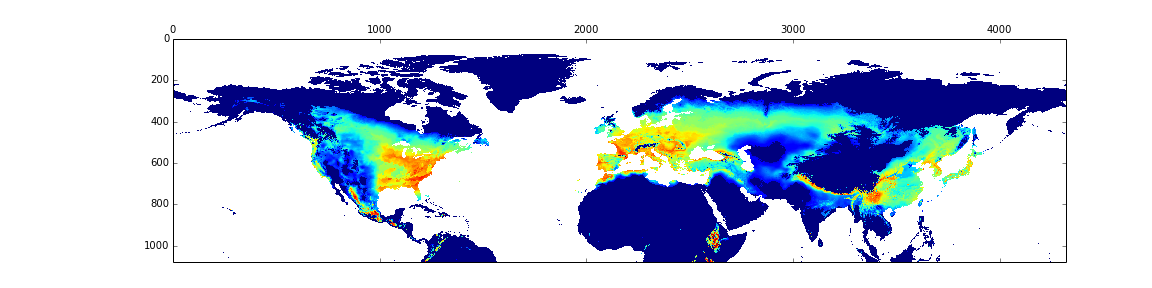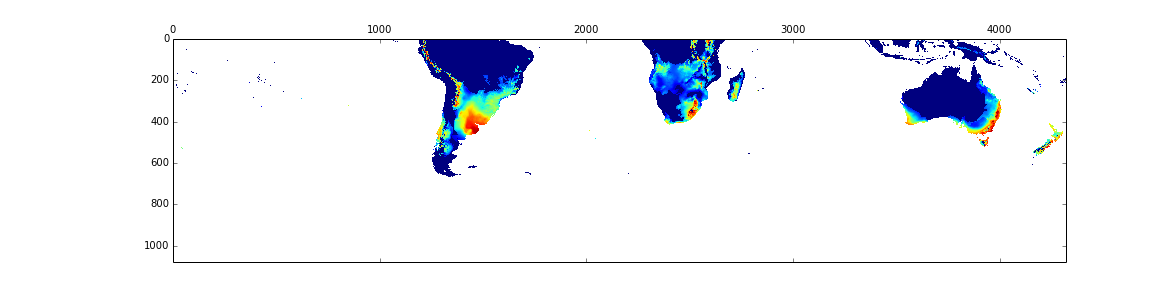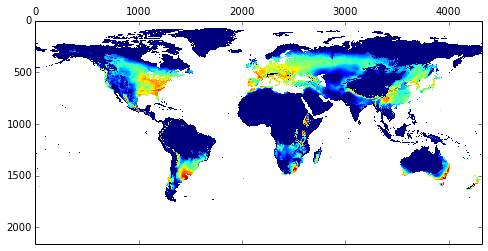## GeoRasters

The `GeoRasters` package is a python module that provides a fast and flexible tool to work with GIS raster files. It provides the GeoRaster class, which makes working with rasters quite transparent and easy. In a way it tries to do for rasters what GeoPandas does for geometries.

It includes tools to

• Merge rasters
• Plot rasters
• Extract information from rasters
• Given a point (lat,lon) find its location in a raster
• Aggregate rasters to lower resolutions
• Align two rasters of different sizes to common area and size
• Get all the geographical information of raster
• Create GeoTiff files easily
• Clip raster using geometries
• Get zonal statistics using geometries
• Spatial analysis tools

## Install

`GeoRasters` can be installed using `pip` or `conda`.

``````pip install git+git://github.com/ozak/georasters.git
pip install georasters
conda install -c conda-forge georasters
conda install -c ozak georasters
``````

You can try it out easily using `conda env` and the provided scripts:

## Requirements

You need to install the following software for `georasters` to work.

• GDAL

## Example Usage: GeoRasters

``````import georasters as gr
import numpy as np

raster = './data/slope.tif'
data = gr.from_file(raster)

# Plot data
data.plot()

# Get some stats
data.mean()
data.sum()
data.std()

# Convert to Pandas DataFrame
df = data.to_pandas()

# Save transformed data to GeoTiff
data2 = data**2
data2.to_tiff('./data2')

# Algebra with rasters
data3 = np.sin(data.raster) / data2
data3.plot()

# Notice that by using the data.raster object,
# you can do any mathematical operation that handles

# Find value at point (x,y) or at vectors (X,Y)
value = data.map_pixel(x,y)
Value = data.map_pixel(X,Y)
``````

## Example Merge GeoRasters:

``````import os
import georasters as gr
import matplotlib.pyplot as plt

DATA = "/path/to/tiff/files"

# Import raster
raster = os.path.join(DATA, 'pre1500.tif')
data = gr.from_file(raster)
(xmin, xsize, x, ymax, y, ysize) = data.geot

# Split raster in two
data1 = gr.GeoRaster(data.raster[:data.shape / 2, :],
data.geot,
nodata_value=data.nodata_value,
projection=data.projection,
datatype=data.datatype)

data2 = gr.GeoRaster(data.raster[data.shape / 2:, :],
(xmin, xsize, x, ymax + ysize * data.shape / 2, y, ysize),
nodata_value=data.nodata_value,
projection=data.projection,
datatype=data.datatype,)

# Plot both parts and save them
plt.figure(figsize=(12, 8))
data1.plot()
plt.savefig(os.path.join(DATA, 'data1.png'), bbox_inches='tight')
````````````plt.figure(figsize=(12,8))
data2.plot()
plt.savefig(os.path.join(DATA,'data2.png'), bbox_inches='tight')
````````````# Generate merged raster

data3 = data1.union(data2)

# Plot it and save the figure
plt.figure(figsize=(12,8))
data3.plot()
plt.savefig(os.path.join(DATA,'data3.png'), bbox_inches='tight')
``````# Example Usage: Other functions

``````import georasters as gr
import numpy as np

# Get info on raster
NDV, xsize, ysize, GeoT, Projection, DataType = gr.get_geo_info(raster)

# Find location of point (x,y) on raster, e.g. to extract info at that location
col, row = gr.map_pixel(x,y,GeoT,GeoT[-1], GeoT,GeoT)
value = data[row,col]

# Agregate raster by summing over cells in order to increase pixel size by e.g. 10
gr.aggregate(data,NDV,(10,10))

# Align two rasters
(alignedraster_o, alignedraster_a, GeoT_a) = gr.align_rasters(raster, raster2, how=np.mean)

# Create GeoRaster
A=gr.GeoRaster(data, GeoT, nodata_value=NDV)

NDV, xsize, ysize, GeoT, Projection, DataType = gr.get_geo_info(raster2)
B=gr.GeoRaster(data2, GeoT, nodata_value=NDV)

# Plot Raster
A.plot()

# Merge both rasters and plot
C=B.merge(A)
C.plot()
``````

## Issues

Find a bug? Report it via Github issues by providing

• a link to download the smallest possible raster and vector dataset necessary to reproduce the error
• python code or command to reproduce the error
• information on your environment: versions of python, gdal and numpy and system memory

Get A Weekly Email With Trending Projects For These Topics
No Spam. Unsubscribe easily at any time.
python (55,539
python3 (1,682
python-3 (213
python-library (156
python2 (136